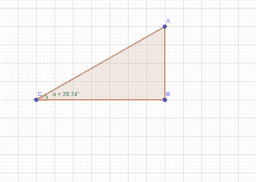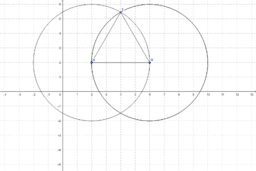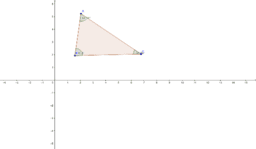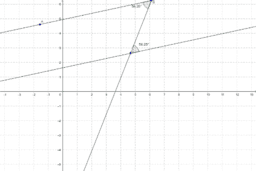Tika Ram Pokhrel

•Ratios

Activity

Tika Ram Pokhrel

•Exploration of Trigonometric Ratios

Book

Tika Ram Pokhrel

•Equilateral Triangle

Activity

Tika Ram Pokhrel

•Central and Circumference angle

Activity

Tika Ram Pokhrel

•Angle Sum of Triangle

Activity

Tika Ram Pokhrel

•Alternative Angles are equal iff Line are Parallel

Activity

Tika Ram Pokhrel